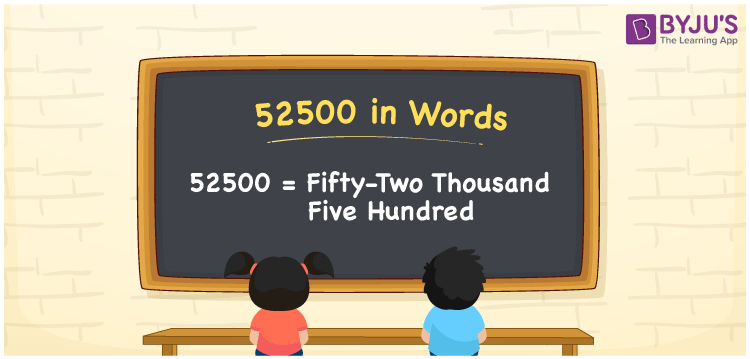# 52500 in Words

52500 in words is Fifty-two thousand Five hundred. If you paid an annual tax of Rs. 52500, you may express it as ” I paid Fifty-two thousand five hundred rupees as annual tax”. In this article, you will understand how to write the cardinal number 52500 in word form easily.

 52500 in words Fifty-two thousand Five hundred Fifty-two thousand Five hundred in Numbers 52500

## 52500 in English words

We generally write numbers in words using the English alphabet. So, we can spell 52500 in English as “Fifty-two thousand Five hundred”.## How to Write 52500 in Words?

The number 52500 has five digits, so we need to create a place value chart with five columns to obtain the place value for all the digits, as shown in the table below.

 Ten thousands Thousands Hundreds Tens Ones 5 2 5 0 0

Here, ones = 0, tens = 0, hundreds = 5, thousands = 2, ten thousands = 5.

Now, expand these numbers, along with the place value.

5 × Ten thousand + 2 × Thousand + 5 × Hundred + 0 × Ten + 0 × One

= 5 × 10000 + 2 × 1000 + 5 × 100 + 0 × 10 + 0 × 1

= 50000 + 2000 + 500

= Fifty thousand + Two thousand + Five hundred

= Fifty-two thousand Five hundred

Thus, 52500 in words = Fifty-two thousand Five hundred.

### Facts About the Number 52500

52500 is a natural number that is the successor of 52499 and the predecessor of 52501.

52500 in words – Fifty-two thousand Five hundred

Is 52500 an odd number? – No

Is 52500 an even number? – Yes

Is 52500 a perfect square number? – No

Is 52500 a perfect cube number? – No

Is 52500 a prime number? – No

Is 52500 a composite number? – Yes

## Frequently Asked Questions on 52500 in Words

Q1

### How do you write 52500 in English words?

In English words, the number 52500 can be written as Fifty-two thousand Five hundred.
Q2

### How to write Rs. 52500 in words on a cheque?

On a cheque, we generally write Rs. 52500 in words as Fifty-two thousand Five hundred rupees only.
Q3

### Express the value in words for 50000 + 2500.

50000 + 2500 = 52500 The value of 50000 + 2500 is 52500, i.e. Fifty-two thousand five hundred.